# Why error message #VALUE! occurs and how to solve it

Uploaded time: February 25, 2022 Difficulty: Beginner# Why error message #VALUE! occurs and how to solve it

The errors in a function or formula will result in calculation errors, and it returns #VALUE!.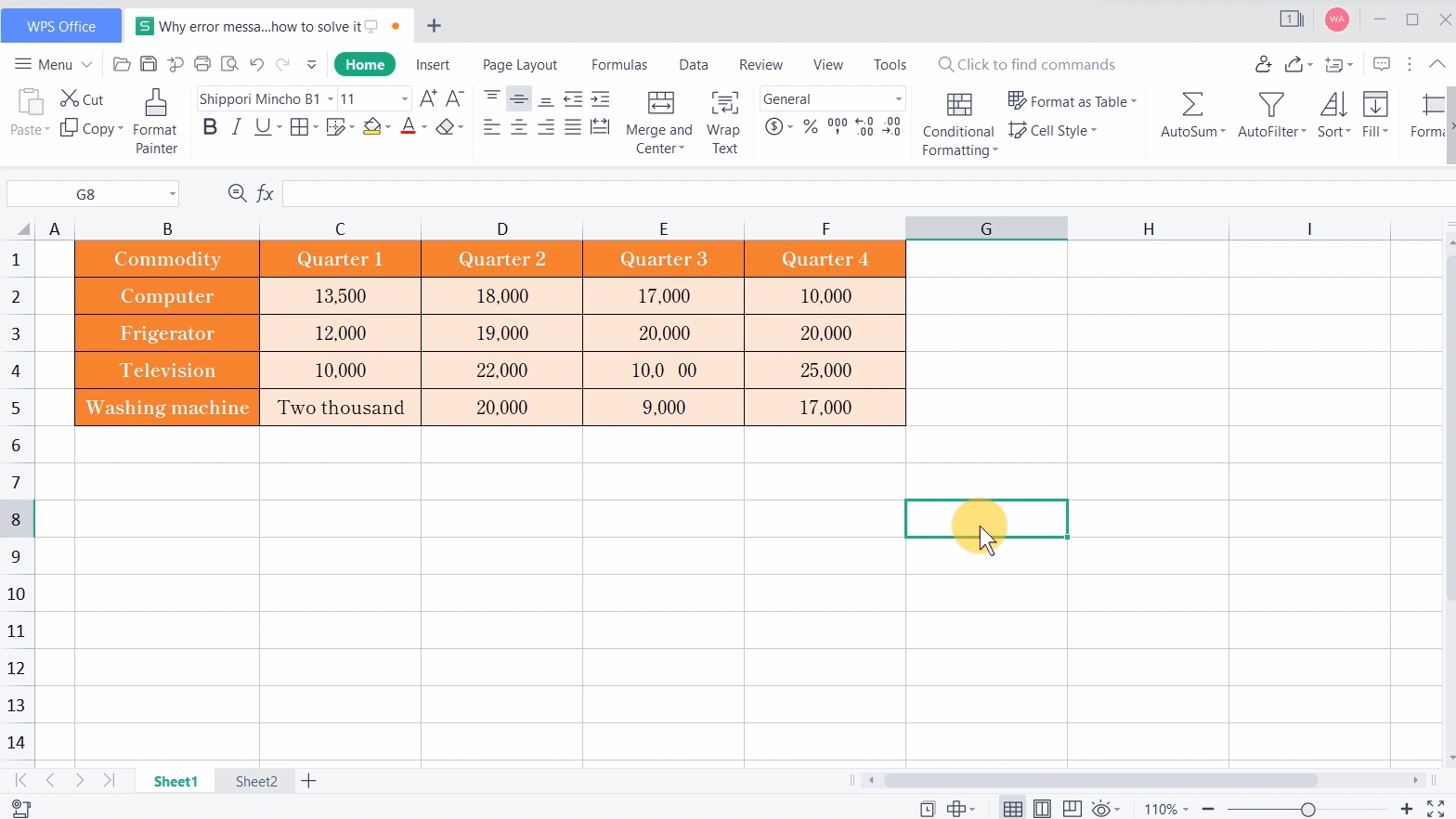The common causes are as follows: incorrect function syntax, the reference cells containing text in the formulas, the reference cells containing a non-character string in the formulas, and the lack of braces in the formulas.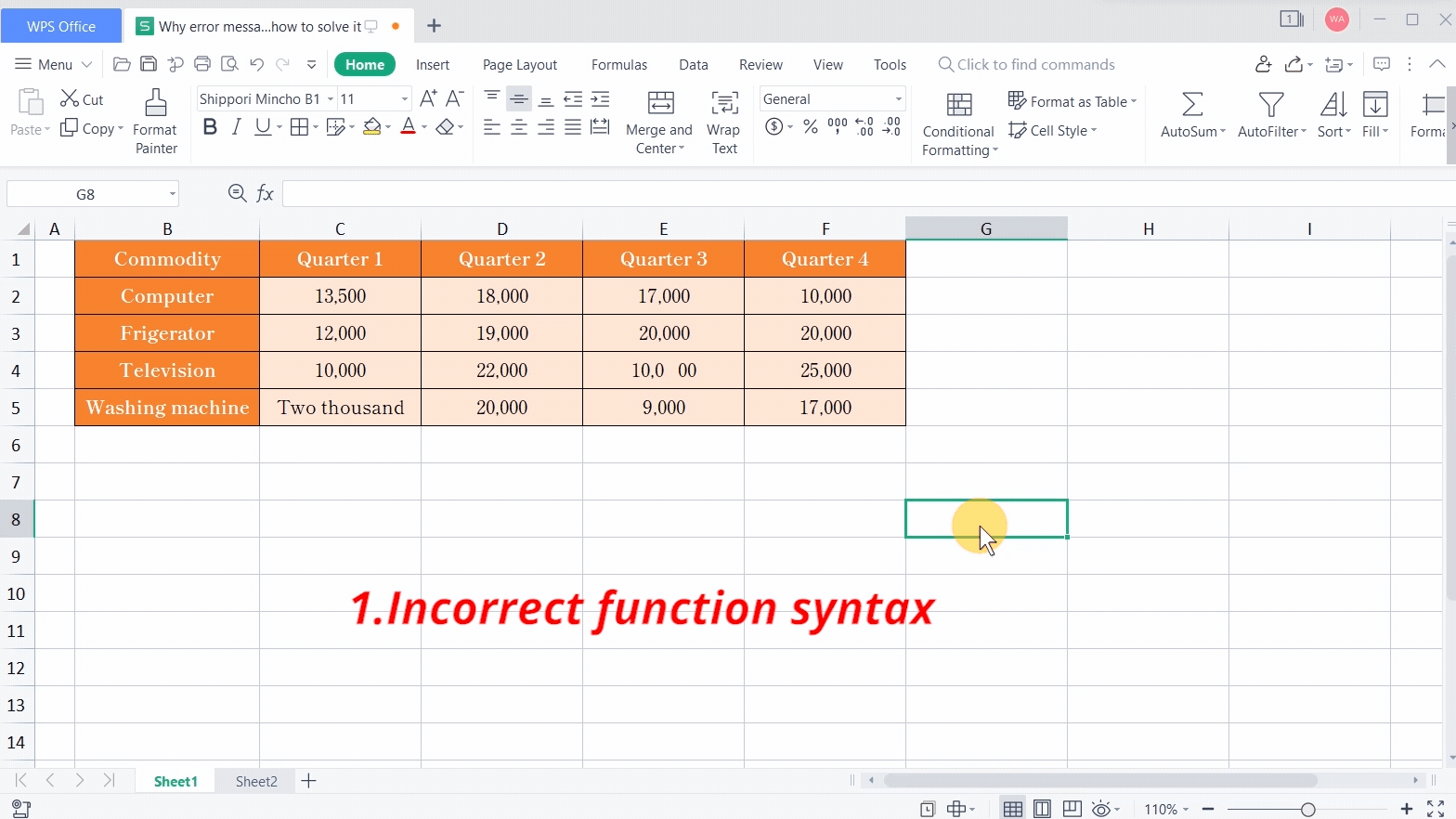Let's go through them one by one.

· Incorrect function syntax.

For example, we want to calculate the difference in computer sales between the first half of this year and the second half. We input =SUM(C2:D2-E2-F2) and press the Enter key. Then it returns #VALUE!.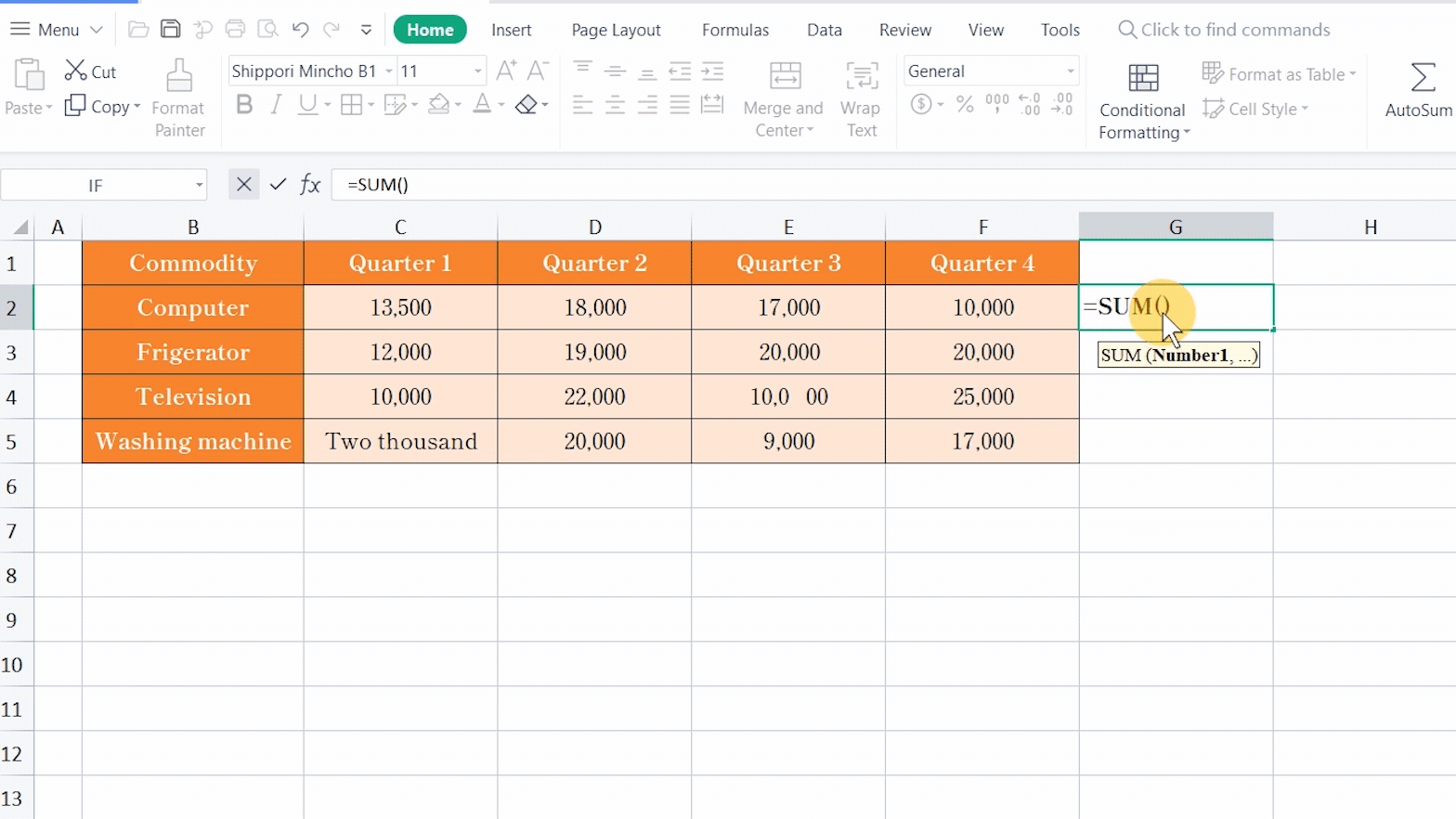That is because C2:D2 refers to continuous cell range reference, and we need a comma to separate discontinuous cell ranges.The solution is to input the cell range correctly. Select G2, and input the formula =SUM(C2:D2, -E2-F2) to return the correct result.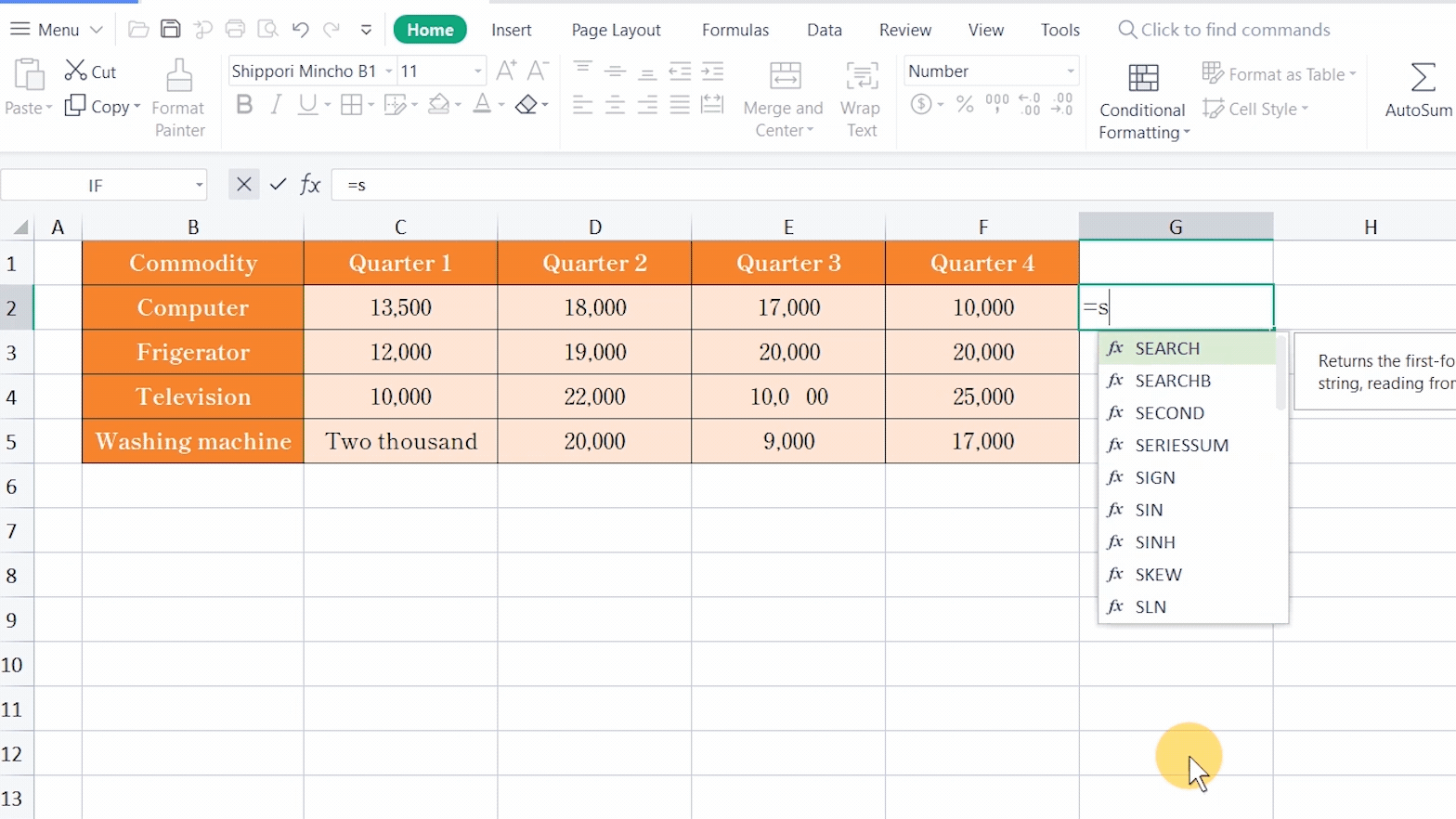· The reference cells containing text in the formulas.

For example, we want to sum up the sales of all commodities in Quarter 1. Input =SUM(C2+C3+C4+C5) in C6, and it returns #VALUE!.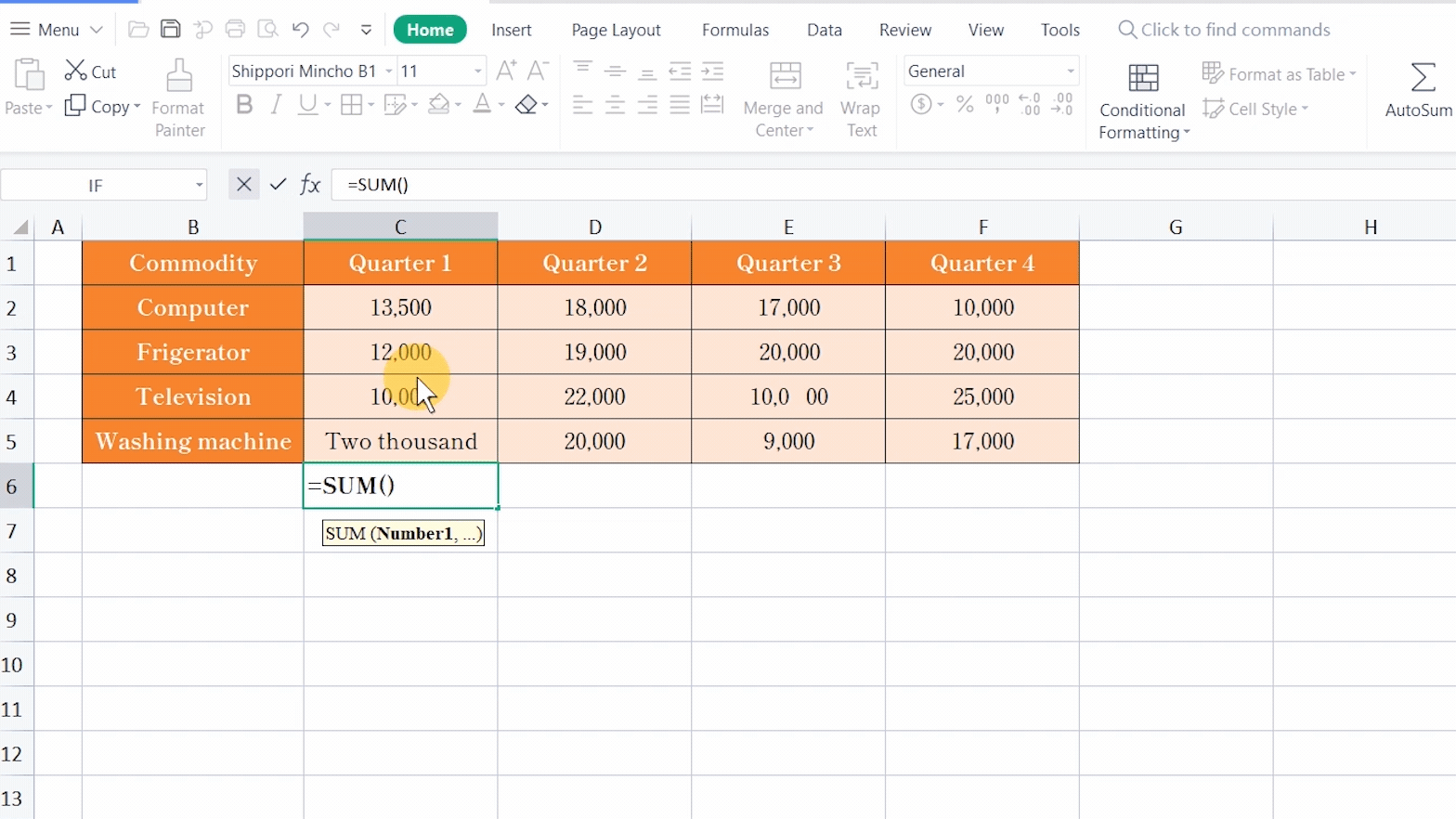That is because any of the reference cells in this formula contain text, like words and special characters.

The solution is to replace the text with numbers. To correct the calculation, select the cell range, use the shortcut key Ctrl+G to navigate to C5, which contains text, and change it to numbers.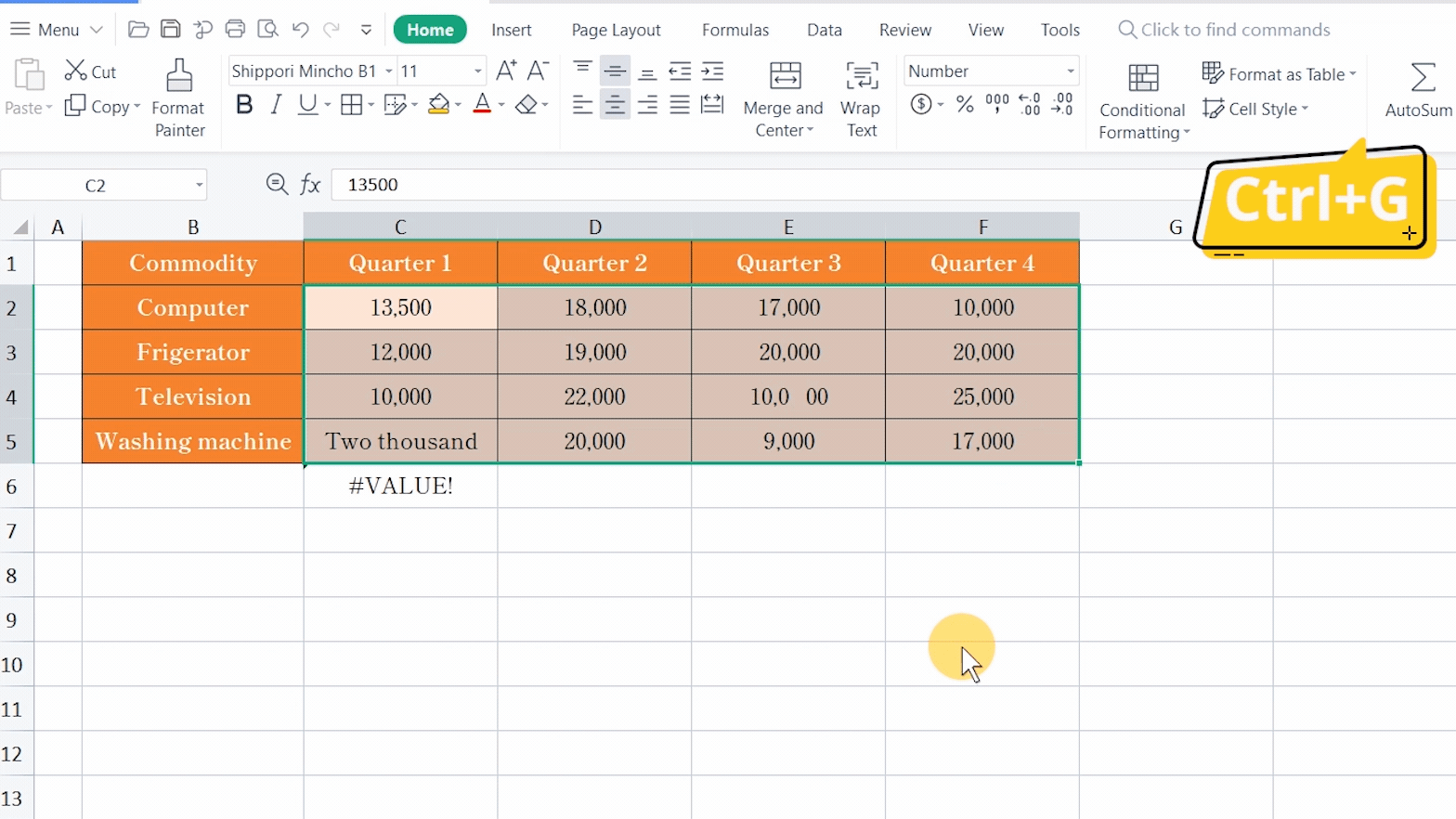· The reference cells containing a non-character string in the formulas.

To sum up the computer sales of the second half of this year, we input the formula =SUM(E4+F4), but it returns #VALUE!.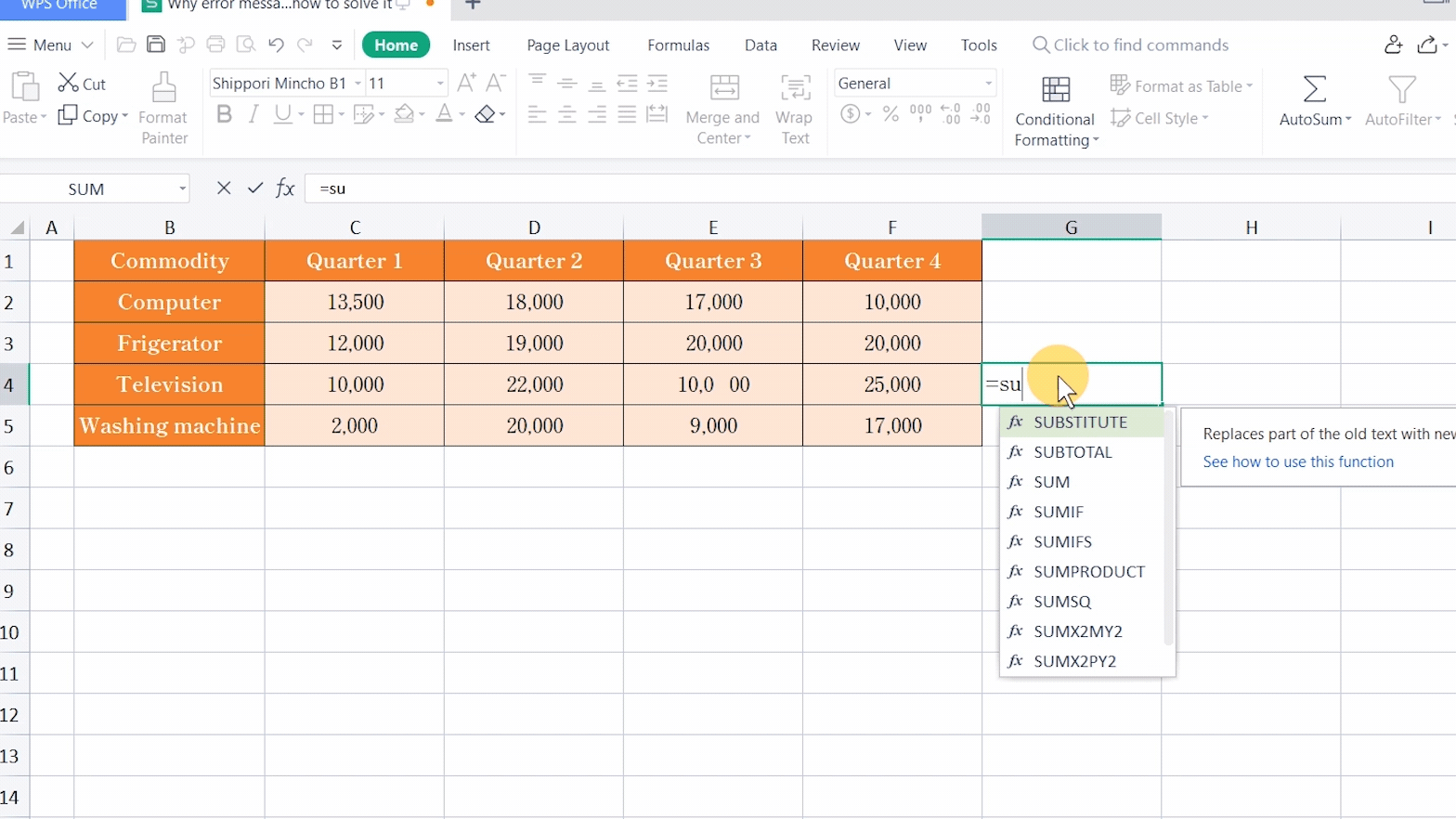That is because the formula references the cells that contain the non-character string, such as E4.

The solution is to delete the non-character string. We can use the Find and Replace function to find it quickly, and its shortcut key is Ctrl+H.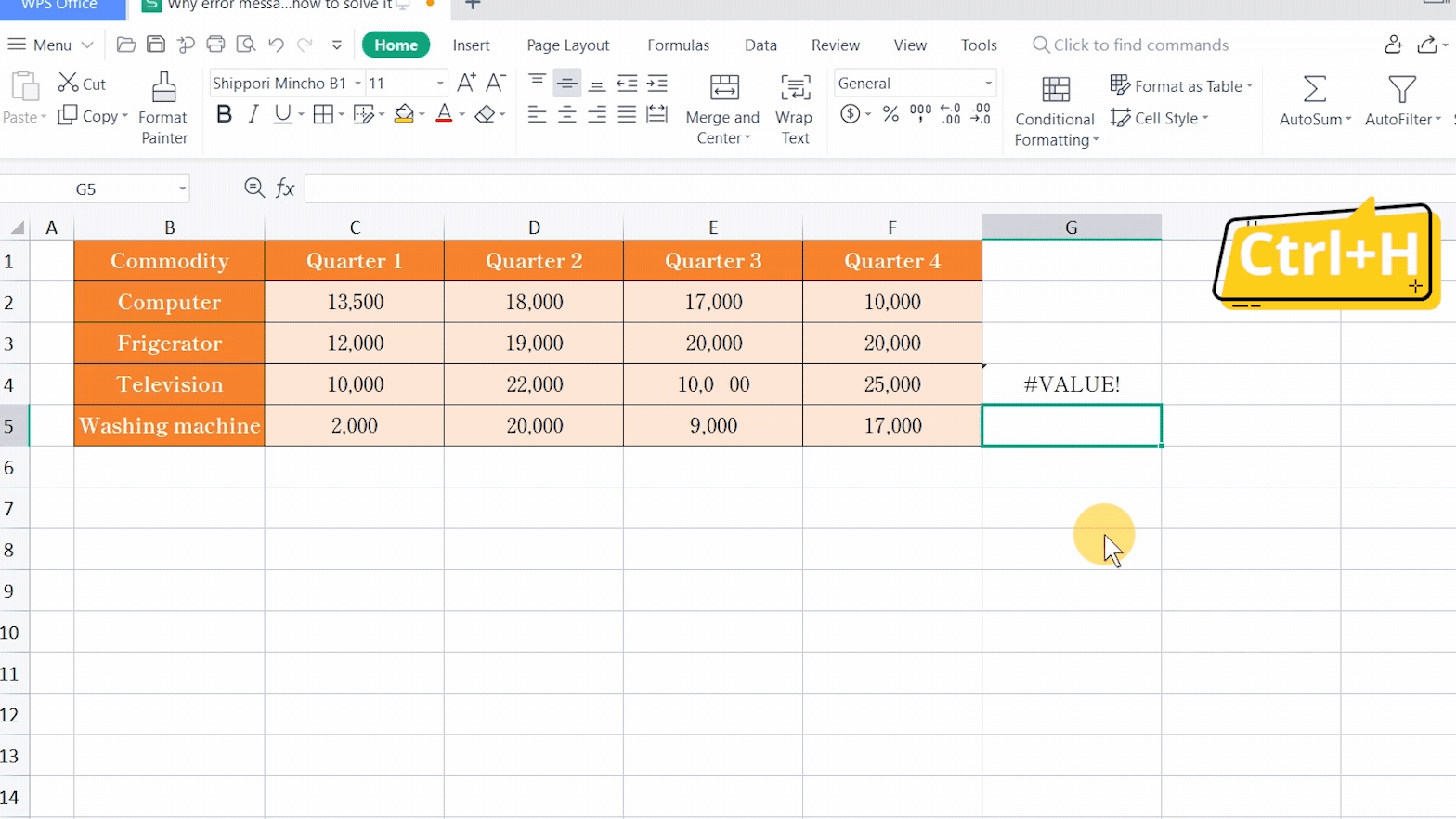· The lack of braces in the formulas.

For example, we want to sum up the stationary sales in the whole table. If we input the formula =SUM(C2:C8*D2:D8) in C10, it returns #VALUE!.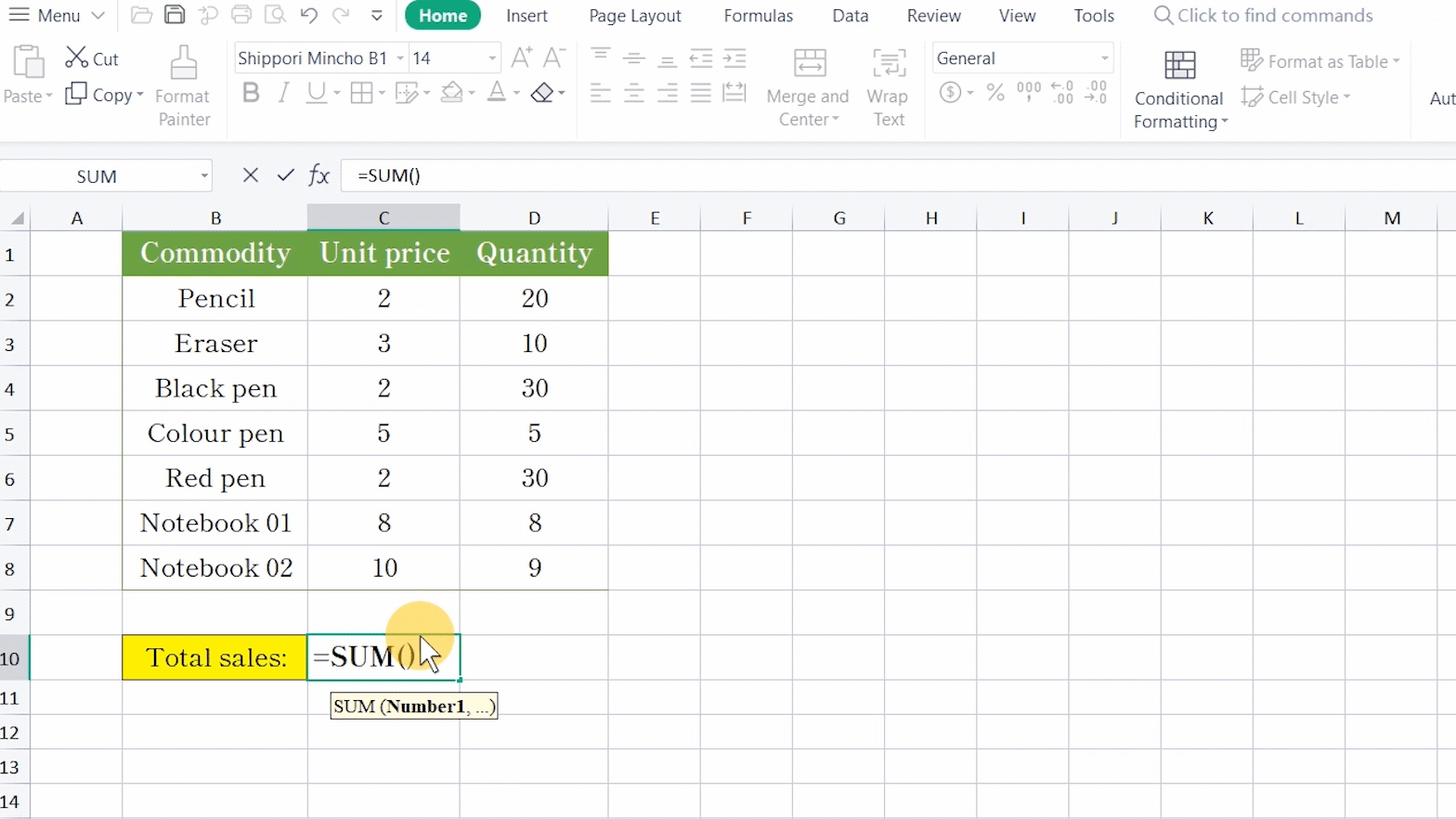That is because of the lack of braces in this formula.

The solution is to press Ctrl+Shift+Enter to use the braces to connect the arrays. Then you can get the result.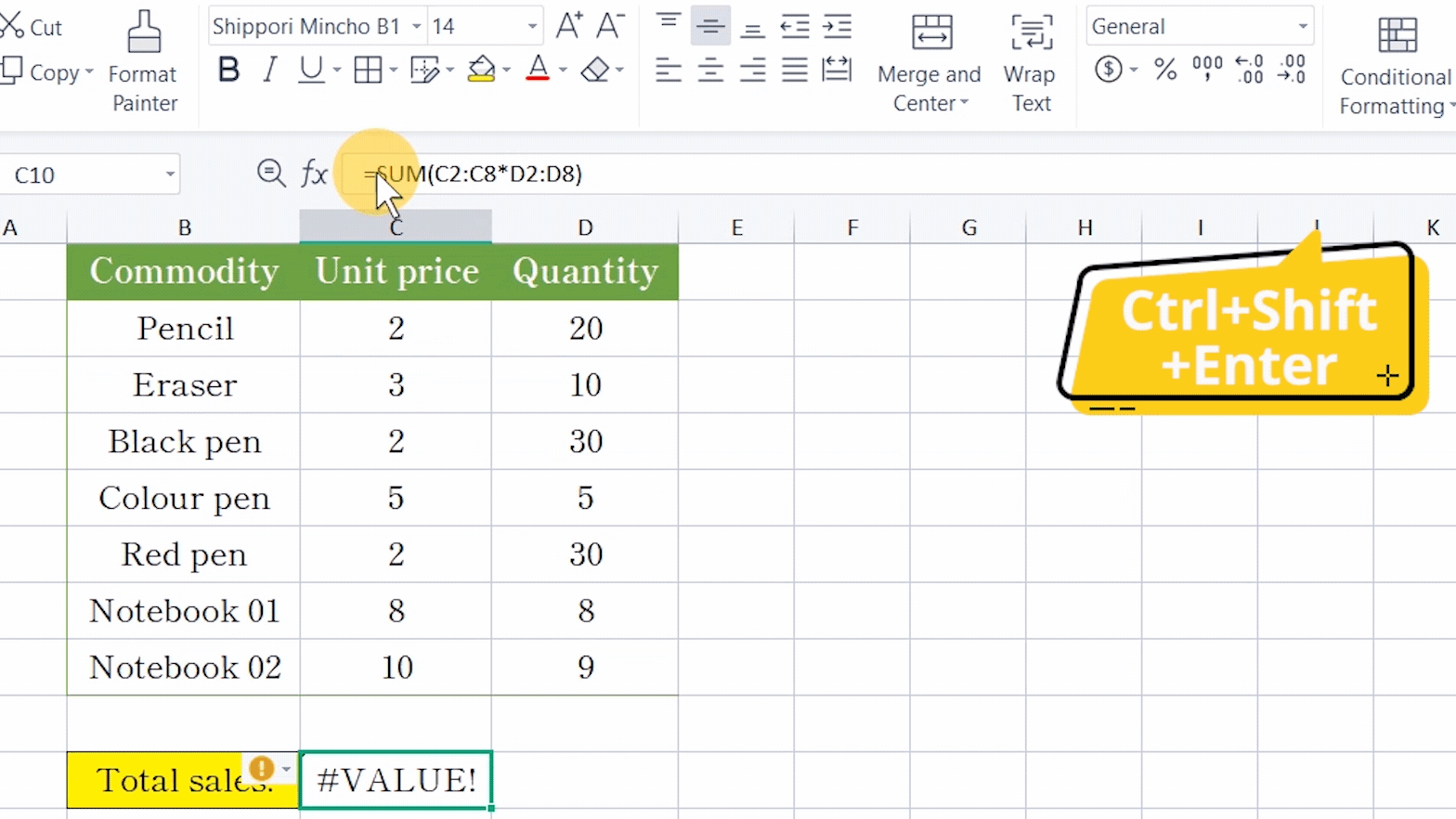These are the common causes of the #VALUE! error. Did you get it?